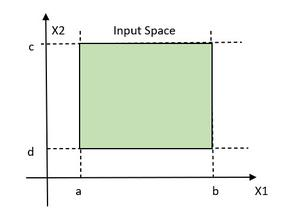Open in App
Not now

# Software Testing – Boundary Value Analysis

• Difficulty Level : Expert
• Last Updated : 27 Mar, 2022

Functional testing is a type of software testing in which the system is tested against the functional requirements of the system. It is conducted to ensure that the requirements are properly satisfied by the application. Functional testing verifies that each function of the software application works in conformance with the requirement and specification. Boundary Value Analysis(BVA) is one of the functional testings.

### Boundary Value Analysis

Boundary Value Analysis is based on testing the boundary values of valid and invalid partitions. The behavior at the edge of the equivalence partition is more likely to be incorrect than the behavior within the partition, so boundaries are an area where testing is likely to yield defects.

It checks for the input values near the boundary that have a higher chance of error. Every partition has its maximum and minimum values and these maximum and minimum values are the boundary values of a partition.

Note:

• A boundary value for a valid partition is a valid boundary value.
• A boundary value for an invalid partition is an invalid boundary value.
• For each variable we check-
• Minimum value.
• Just above the minimum.
• Nominal Value.
• Just below Max value.
• Max value.

Example: Consider a system that accepts ages from 18 to 56.

Valid Test cases: Valid test cases for the above can be any value entered greater than 17 and less than 57.

• Enter the value- 18.
• Enter the value- 19.
• Enter the value- 37.
• Enter the value- 55.
• Enter the value- 56.

Invalid Testcases: When any value less than 18 and greater than 56 is entered.

• Enter the value- 17.
• Enter the value- 57.

Single Fault Assumption: When more than one variable for the same application is checked then one can use a single fault assumption. Holding all but one variable to the extreme value and allowing the remaining variable to take the extreme value. For n variable to be checked:

Maximum of 4n+1 test cases

Problem: Consider a Program for determining the Previous Data.

Input: Day, Month, Year with valid ranges as-

1 ≤ Month≤12
1 ≤ Day ≤31
1900 ≤ Year ≤ 2000

Design Boundary Value Test Cases.

Solution: Taking the year as a Single Fault Assumption i.e. year will be having values varying from 1900 to 2000 and others will have nominal values.

Taking Day as Single Fault Assumption i.e. Day will be having values varying from 1 to 31 and others will have nominal values.

Taking Month as Single Fault Assumption i.e. Month will be having values varying from 1 to 12 and others will have nominal values.

For the n variable to be checked Maximum of 4n + 1 test case will be required. Therefore, for n = 3, the maximum test cases are-

4 × 3 + 1 =13

The focus of BVA: BVA focuses on the input variable of the function. Let’s define two variables X1 and X2, where X1 lies between a and b and X2 lies between c and d.Showing legitimate domain

The idea and motivation behind BVA are that errors tend to occur near the extremes of the variables. The defect on the boundary value can be the result of countless possibilities.

Typing of Languages: BVA is not suitable for free-form languages such as COBOL and FORTRAN, These languages are known as weakly typed languages. This can be useful and can cause bugs also.

PASCAL, ADA is the strongly typed language that requires all constants or variables defined with an associated data type.

Limitation of Boundary Value Analysis:

• It works well when the product is under test.
• It cannot consider the nature of the functional dependencies of variables.
• BVA is quite rudimentary.

### Equivalence Partitioning

It is a type of black-box testing that can be applied to all levels of software testing. In this technique, input data are divided into the equivalent partitions that can be used to derive test cases-

• In this input data are divided into different equivalence data classes.
• It is applied when there is a range of input values.

Example: Below is the example to combine Equivalence Partitioning and Boundary Value.

Consider a field that accepts a minimum of 6 characters and a maximum of 10 characters. Then the partition of the test cases ranges 0 – 5, 6 – 10, 11 – 14.

Why Combine Equivalence Partitioning and Boundary Analysis Testing: Following are some of the reasons why to combine the two approaches:

• In this test cases are reduced into manageable chunks.
• The effectiveness of the testing is not compromised on test cases.
• Works well with a large number of variables.

My Personal Notes arrow_drop_up
Related Articles